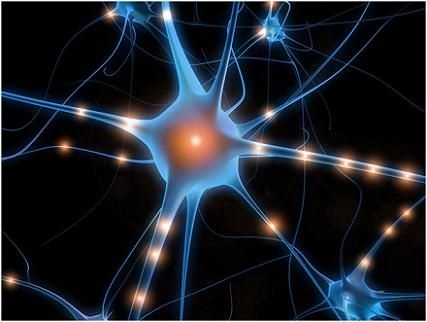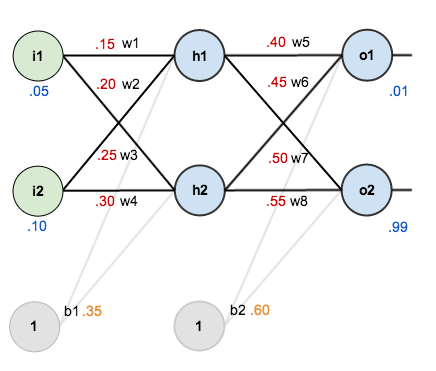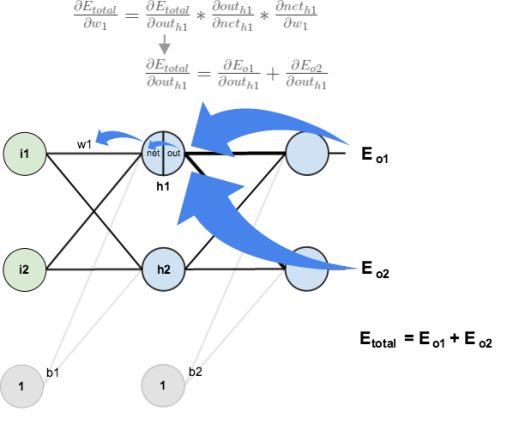• 神经网络
• 理解神经元的工作原理
• 简单神经元模型
• 神经网络模型
• 多层神经网络
• 反向传导（BP）算法思路
• 定义损失函数
• 随机初始化参数
• 计算残差
• BP算法的一次实例更新迭代
• 第一步：前馈传导
• 第二步：反向传导
• 参考文献

# 神经网络

## 理解神经元的工作原理

“神经网络”一词起源于早先模拟（mimic）人类大脑的研究中，而发展到今天的“神经网络”早就已经是一个庞大的交叉学科领域，当然本文讨论的范围在机器学习与神经网络交叉的部分。在进行正式讨论之前，我们有必要先了解一些神经网络的前世今生。## 简单神经元模型## 神经网络模型

“…our intuitive judgment that the extension (to multilayer systems) is sterile”

“···我们最先对多层神经网络系统的判断是徒劳的”## 多层神经网络（注：系数$W_{ij}^{(l)}$表示第$l$层的$j$个单元与第$(l+1)$层第$i$单元的权重

\begin{equation}\begin{split}
z^{(l+1)} = W^{(l)}x + b^{(l)} \
a^{(l+1)} = f(z^{(l+1)})
\end{split}\end{equation}

# 反向传导（BP）算法思路

1. 前馈传导运算，得到各输出层的输出；
2. 从输出层反推进行反向传导计算，利用梯度下降算法更新参数

## 计算残差

\begin{equation}\begin{split}
\delta_i^{(nl)} &= \frac {\partial} {\partial{z_i^{(nl)}}} \frac{1}{2} (y_i - a_j^{(nl)})^2 \
&=\frac {\partial} {\partial{z_i^{(nl)}}} \frac{1}{2} (y_i - f(z_j^{(nl)}))^2 \
& =-(y_i-f(z_i^{(nl)}))\cdot f’(z_i^{(nl)})\
& =-(y_i-a_i^{(nl)}) \cdot f’(z_i^{(nl)})\
\label{residual0}
\end{split}\end{equation}

\begin{equation}\begin{split}
\delta_i^{(nl-1)} &= \frac {\partial} {\partial{z_i^{(nl-1)}}} \frac{1}{2} \sum (y_i - a_j^{(nl)})^2 \
&=\sum \frac {\partial} {\partial{z_i^{(nl-1)}}} \frac{1}{2} (y_i - f(z_j^{(nl)}))^2 \
& =\sum -(y_i-f(z_i^{(nl)}))\cdot f’(z_j^{(nl)}) \cdot \frac{\partial z_j^{(nl)}}{\partial z_j^{(nl-1)}}\
& =\sum \delta_j^{(nl)} \frac{\partial z_j^{(nl)}} {\partial z_j^{(nl-1)}}\
& =(\sum \delta_j^{(nl)} W^{nl-1})f’(z_i^{(nl-1)})\
\label{residual1}
\end{split}\end{equation}

# BP算法的一次实例更新迭代

## 第一步：前馈传导## 第二步：反向传导$W{11}^{(1)}$影响到$a_1^{(2)}$的输出，而$a_1^{(2)}$接受来自两个输出$a_1^{(3)}$和$a_2^{(3)}$的误差，所以我们先将偏导数进行到$a_1^{(2)}$，实际上是求解$\sum \delta_j^{(3)} W{ij}^{2}$

\begin{split}
\sum \deltaj^{(3)} W{ij}^{(2)} &= \frac {\partial{E_T}} {\partial{a_1^{(3)}}} \frac{\partial{a_1^{(3)}}}{\partial {a_1^{(2)}}} + \frac {\partial{E_T}} {\partial{a_2^{(3)}}} \frac{\partial{a_2^{(3)}}}{\partial {a_1^{(2)}}} \
&=0.13850.4+(-0.0381)0.5 \
&=0.0538+(-0.0190)\&=0.0348 \
\end{split}

\begin{equation}\begin{split}
\delta1^{(2)} = (\sum \delta_j^{(3)} W{ij}^{(2)}) \cdot f’(z1^{2})\
\label{key}
\end{split}\end{equation}

# 参考文献

1. 反向传播算法Python代码 | 我爱自然语言处理
2. 神经网络 - Ufldl
3. 神經元》. 2016. 维基百科，自由的百科全书
4. 微软实现深层神经网络重大技术突破 - 微软亚洲研究院
5. CS231n Convolutional Neural Networks for Visual Recognition
6. Neural Networks
7. 《机器学习》. 周志华
8. 《统计学习方法》. 李航
9. 《The Elements of Statistical Learning》Jerome H. Friedman, Robert Tibshirani, and Trevor Hastie
10. A Step by Step Backpropagation Example – Matt Mazur
------本文结束，欢迎收藏本站、分享、评论或联系作者！------

(>看完记得五星好评哦亲<)
0%